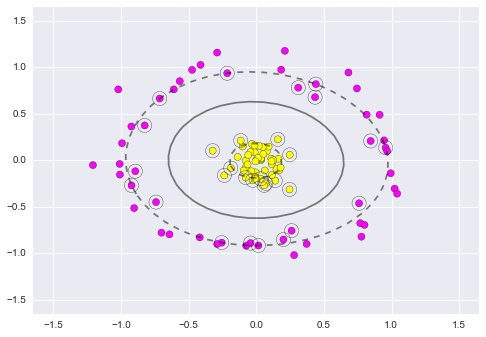scikit-learn-svm¶

Credits: Forked from PyCon 2015 Scikit-learn Tutorial by Jake VanderPlas

• Support Vector Machine Classifier
• Support Vector Machine with Kernels Classifier
In :
%matplotlib inline
import numpy as np
import matplotlib.pyplot as plt
import seaborn;
from sklearn.linear_model import LinearRegression
from scipy import stats
import pylab as pl

seaborn.set()

Support Vector Machine Classifier¶

Support Vector Machines (SVMs) are a powerful supervised learning algorithm used for classification or for regression. SVMs draw a boundary between clusters of data. SVMs attempt to maximize the margin between sets of points. Many lines can be drawn to separate the points above:

In :
from sklearn.datasets.samples_generator import make_blobs
X, y = make_blobs(n_samples=50, centers=2,
random_state=0, cluster_std=0.60)

xfit = np.linspace(-1, 3.5)
plt.scatter(X[:, 0], X[:, 1], c=y, s=50, cmap='spring')

# Draw three lines that couple separate the data
for m, b, d in [(1, 0.65, 0.33), (0.5, 1.6, 0.55), (-0.2, 2.9, 0.2)]:
yfit = m * xfit + b
plt.plot(xfit, yfit, '-k')
plt.fill_between(xfit, yfit - d, yfit + d, edgecolor='none', color='#AAAAAA', alpha=0.4)

plt.xlim(-1, 3.5);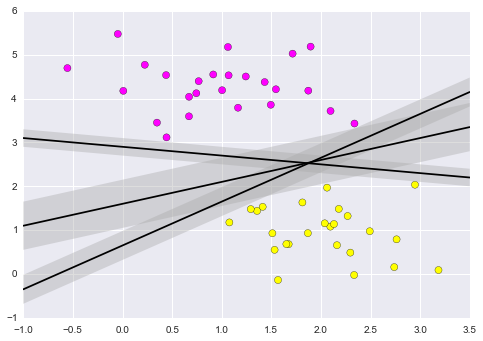Fit the model:

In :
from sklearn.svm import SVC
clf = SVC(kernel='linear')
clf.fit(X, y)
Out:
SVC(C=1.0, cache_size=200, class_weight=None, coef0=0.0, degree=3, gamma=0.0,
kernel='linear', max_iter=-1, probability=False, random_state=None,
shrinking=True, tol=0.001, verbose=False)

Plot the boundary:

In :
def plot_svc_decision_function(clf, ax=None):
"""Plot the decision function for a 2D SVC"""
if ax is None:
ax = plt.gca()
x = np.linspace(plt.xlim(), plt.xlim(), 30)
y = np.linspace(plt.ylim(), plt.ylim(), 30)
Y, X = np.meshgrid(y, x)
P = np.zeros_like(X)
for i, xi in enumerate(x):
for j, yj in enumerate(y):
P[i, j] = clf.decision_function([xi, yj])
# plot the margins
ax.contour(X, Y, P, colors='k',
levels=[-1, 0, 1], alpha=0.5,
linestyles=['--', '-', '--'])

In the following plot the dashed lines touch a couple of the points known as support vectors, which are stored in the support_vectors_ attribute of the classifier:

In :
plt.scatter(X[:, 0], X[:, 1], c=y, s=50, cmap='spring')
plot_svc_decision_function(clf)
plt.scatter(clf.support_vectors_[:, 0], clf.support_vectors_[:, 1],
s=200, facecolors='none');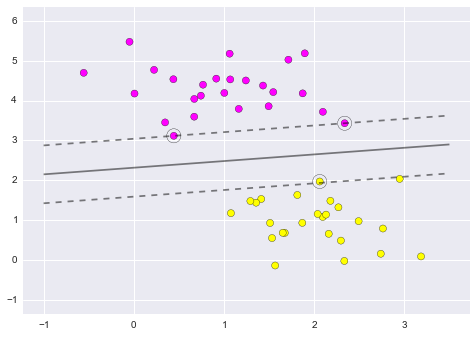Use IPython's interact functionality to explore how the distribution of points affects the support vectors and the discriminative fit:

In :
from IPython.html.widgets import interact

def plot_svm(N=100):
X, y = make_blobs(n_samples=200, centers=2,
random_state=0, cluster_std=0.60)
X = X[:N]
y = y[:N]
clf = SVC(kernel='linear')
clf.fit(X, y)
plt.scatter(X[:, 0], X[:, 1], c=y, s=50, cmap='spring')
plt.xlim(-1, 4)
plt.ylim(-1, 6)
plot_svc_decision_function(clf, plt.gca())
plt.scatter(clf.support_vectors_[:, 0], clf.support_vectors_[:, 1],
s=200, facecolors='none')

interact(plot_svm, N=[10, 200], kernel='linear');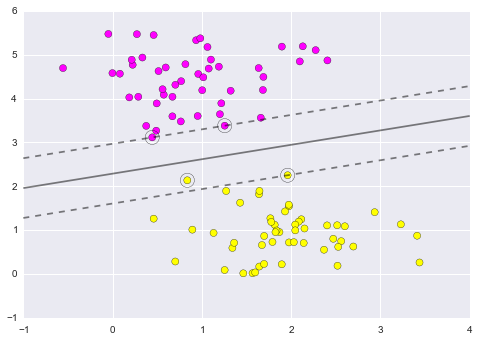Support Vector Machine with Kernels Classifier¶

Kernels are useful when the decision boundary is not linear. A Kernel is some functional transformation of the input data. SVMs have clever tricks to ensure kernel calculations are efficient. In the example below, a linear boundary is not useful in separating the groups of points:

In :
from sklearn.datasets.samples_generator import make_circles
X, y = make_circles(100, factor=.1, noise=.1)

clf = SVC(kernel='linear').fit(X, y)

plt.scatter(X[:, 0], X[:, 1], c=y, s=50, cmap='spring')
plot_svc_decision_function(clf);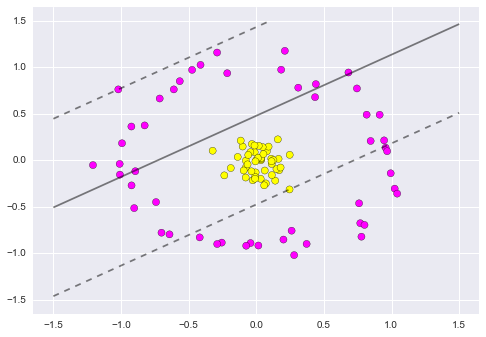A simple model that could be useful is a radial basis function:

In :
r = np.exp(-(X[:, 0] ** 2 + X[:, 1] ** 2))

from mpl_toolkits import mplot3d

def plot_3D(elev=30, azim=30):
ax = plt.subplot(projection='3d')
ax.scatter3D(X[:, 0], X[:, 1], r, c=y, s=50, cmap='spring')
ax.view_init(elev=elev, azim=azim)
ax.set_xlabel('x')
ax.set_ylabel('y')
ax.set_zlabel('r')

interact(plot_3D, elev=[-90, 90], azip=(-180, 180));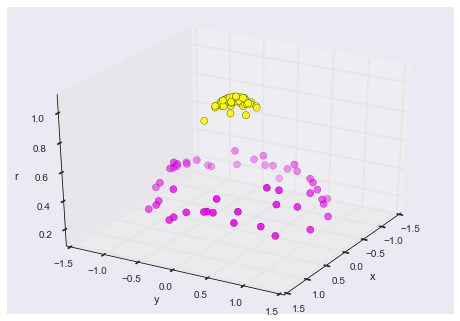In three dimensions, there is a clear separation between the data. Run the SVM with the rbf kernel:

In :
clf = SVC(kernel='rbf')
clf.fit(X, y)

plt.scatter(X[:, 0], X[:, 1], c=y, s=50, cmap='spring')
plot_svc_decision_function(clf)
plt.scatter(clf.support_vectors_[:, 0], clf.support_vectors_[:, 1],
s=200, facecolors='none');